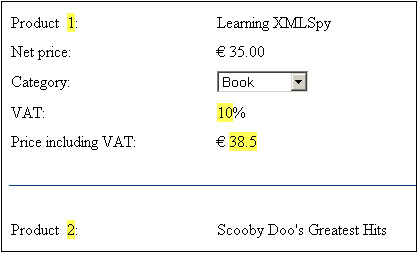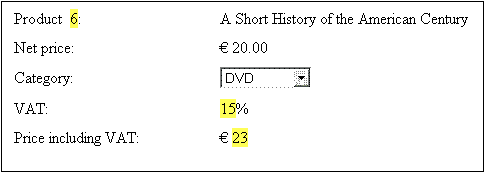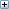# Altova StyleVision 2020 Basic Edition

 Example: An Invoice Scroll Home Prev Top Next More

The SimpleInvoice.sps example in the (My) Documents folder, C:\Documents and Settings\<username>\My Documents\Altova\StyleVision2020\StyleVisionExamples\Tutorial\Auto-Calculations\, demonstrates how Auto-Calculations can be used for the following purposes:

Counting nodes

Selecting a node based on input from the Authentic View user

Creating complex calculations

In the example file, the Auto-Calculations have been highlighted with a yellow background color (see screenshot below).

## Counting nodes

In the SimpleInvoice example, each product in the list is numbered according to its position in the list of products that a customer has ordered (Product 1, Product 2, etc). This numbering is achieved with an Auto-Calculation (screenshot below).In this particular case, the XPath expression position() would suffice to obtain the correct numbering. Another useful way to obtain the position of a node is to count the number of preceding siblings and add one. The XPath expression would be: count(preceding-sibling::Product)+1. The latter approach could prove useful in contexts where the position() function is difficult to use or cannot be used. You can test this Auto-Calculation in the example file by deleting products, and/or adding and deleting new products.

## Selecting a node based on user input

In the SimpleInvoice example, the product category (Book, CD, DVD, or Electronics) is contained in the //Product/Category node and is displayed in a combo box. This selection is entered in the //Product/Category node in the XML document. An Auto-Calculation then uses this value to reference a "lookup table" in the XML document and identify the node holding the VAT percentage for this product category. The XPath expression of this Auto-Calculation is:

for \$i in Category return /Invoice/Categories/Category[. = \$i]/@rate.

The VAT percentage is displayed at the Auto-Calculation location in the output. In the Invoices example, the lookup table is stored in the same XML document as the invoice data. However, such a table can also be stored in a separate document, in which case it would be accessed using the doc() function of XPath 2.0. Notice that the VAT value of different products are different (Book=10%; CD=15%; DVD=15%; Electronics=20%); they have been calculated by the Auto-Calculation.

## Creating a complex Auto-Calculation

The VAT percentage, obtained by the Auto-Calculation described above, is required to calculate the gross price (net price + VAT amount) of each product. The formula to use would be derived as follows:

Gross Price = Net Price + VAT-amount

Since VAT-amount = Net Price * VAT-percentage div 100

Gross Price = Net Price + (Net Price * VAT-percentage div 100)

The net price of a product is obtained from the PriceNet node. The VAT percentage is calculated by an Auto-Calculation as described above; it is not contained in any node. Since this value cannot be obtained directly from a node, it must be re-calculated in the gross price Auto-Calculation. The XPath expression to do this would be:

for \$i in Category return PriceNet + (PriceNet *(/Invoice/Categories/Category[. = \$i]/@rate) div 100)

The XPath expression can be viewed and edited in the Properties window. You can test the Auto-Calculation for the gross price by changing, in the XML file and then re-loading the SPS, either the price or product category of any product. Notice that the gross price (price including VAT) of the product also changes.See also